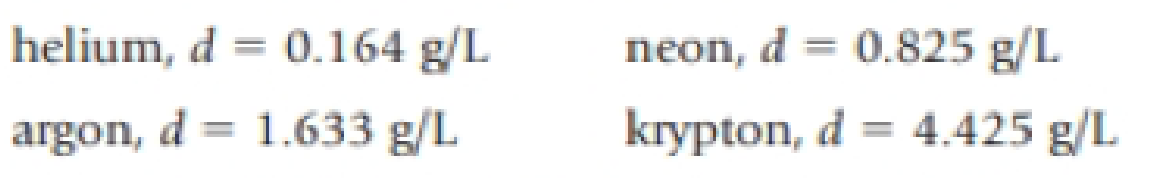Chapter 1, Problem 53GQ

Chapter
Section
Textbook Problem

Four balloons are each filled with a different gas, each having a different density:If the density of dry air is 1.12 g/L, which balloon or balloons float in air?

Interpretation Introduction

Interpretation:

The balloons that float in air has to be given.

Concept introduction:

Density:

Density can be defined as property that links mass of an object to its volume.  It derived quantity and its unit is gml-1 for liquids and gcm-3 for solid.  The density of material can be calculated with the help of formula,

Density=Mass(g)Volume(mlorcm3)

Explanation

The density of dry air is 1.12g/L,

Still sussing out bartleby?

Check out a sample textbook solution.

See a sample solution

The Solution to Your Study Problems

Bartleby provides explanations to thousands of textbook problems written by our experts, many with advanced degrees!

Get Started

Find more solutions based on key concepts